Function Repository Resource:

# IncompletePFD

Give the incomplete partial fraction decomposition of a rational function

Contributed by: Daniel Lichtblau
 ResourceFunction["IncompletePFD"][num,den,x] returns the incomplete partial fraction decomposition of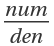.

## Details and Options

For a rational function of the form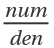in the variable x, where den factors as d1e1dmem, the incomplete partial fraction decomposition is the unique way of rewriting as a sum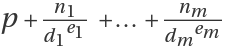, where den is factored into a product of powers of irreducible polynomials.
In the special case where all exponents are equal to 1, this is the same as the full partial fraction decomposition.
ResourceFunction["IncompletePFD"] takes a Modulus option that can affect the factorization.
ResourceFunction["IncompletePFD"] also supports an Extension option that gives algebraic numbers to be allowed in the factorization.

## Examples

### Basic Examples (1)

Find the incomplete partial fraction decomposition for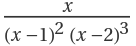:

 In:=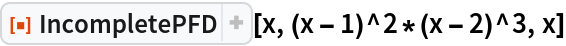Out=### Options (2)

Find the incomplete partial fraction decomposition formodulo 5:

 In:=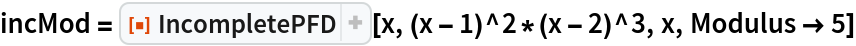Out=Check the result is equivalent to the rational function:

 In:=Out=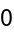The rational function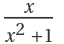does not have a nontrivial decomposition over the rationals:

 In:=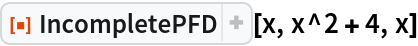Out=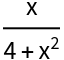The denominator factors over the Gaussian integers, so providing that as an extension gives a decomposition over linear denominators:

 In:=Out=### Properties and Relations (6)

Apart is similar to IncompletePFD, but it does a full decomposition over the rationals. First define a numerator and denominator:

 In:=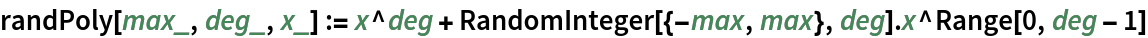In:=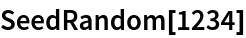In:=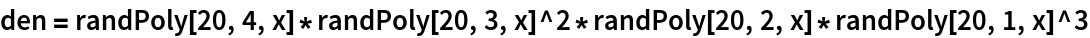Out=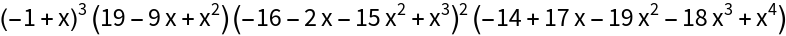In:=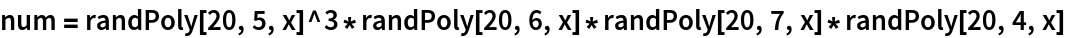Out=Compute the incomplete partial fraction decomposition:

 In:=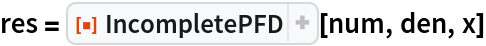Out=Apart gives a mathematically equivalent result :

 In:=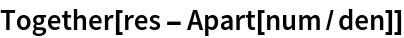Out=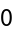For high degrees, IncompletePFD can be notably faster than Apart:

 In:=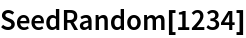In:=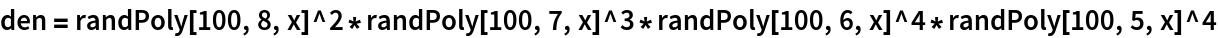Out=In:=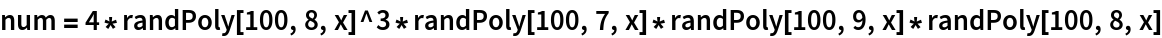Out=In:=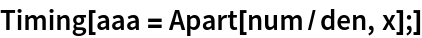Out=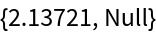In:=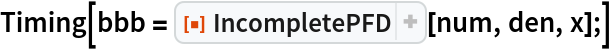Out=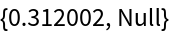Check that the denominators are the expected factors to the correct powers:

 In:=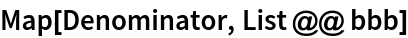Out=Check that the result is equivalent to the rational function num/den:

 In:=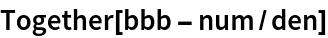Out=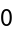### Possible Issues (1)

IncompletePFD is intended for polynomials with exact coefficients and may give a result that is deficient due to numerical instability of the method when given input with approximate coefficients:

 In:=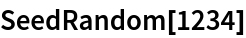In:=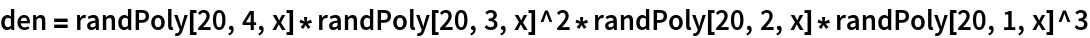Out=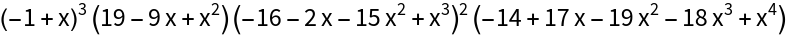In:=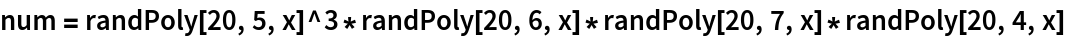Out=In:=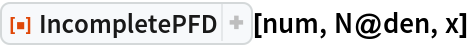Out=## Version History

• 1.0.0 – 16 August 2019

## Author Notes

This function uses a divide-and-conquer approach. It could be more efficient and divide based on keeping total degrees as similar as possible to one another. The inexact coefficient case is, at best, a jury-rig. Improvements would include different functions to handle the extended GCDs and quotient/remainder uses.